Documentation

interpolateStrain

Interpolate strain at arbitrary spatial locations

Description

example

intrpStrain = interpolateStrain(structuralresults,xq,yq) returns the interpolated strain values at the 2-D points specified in xq and yq. For transient and frequency-response structural models, interpolateStrain interpolates strain for all time- or frequency-steps, respectively.

example

intrpStrain = interpolateStrain(structuralresults,xq,yq,zq) uses the 3-D points specified in xq, yq, and zq.

example

intrpStrain = interpolateStrain(structuralresults,querypoints) uses the points specified in querypoints.

Examples

collapse all

Create a structural analysis model for a plane-strain problem.

structuralmodel = createpde('structural','static-planestrain');

Include the square geometry in the model. Plot the geometry.

geometryFromEdges(structuralmodel,@squareg);
pdegplot(structuralmodel,'EdgeLabels','on')
axis equalSpecify the Young's modulus and Poisson's ratio.

structuralProperties(structuralmodel,'PoissonsRatio',0.3, ...
'YoungsModulus',210E3);

Specify the x-component of the enforced displacement for edge 1.

structuralBC(structuralmodel,'XDisplacement',0.001,'Edge',1);

Specify that edge 3 is a fixed boundary.

structuralBC(structuralmodel,'Constraint','fixed','Edge',3);

Generate a mesh and solve the problem.

generateMesh(structuralmodel);
structuralresults = solve(structuralmodel);

Create a grid and interpolate the x- and y-components of the normal strain to the grid.

v = linspace(-1,1,101);
[X,Y] = meshgrid(v);
intrpStrain = interpolateStrain(structuralresults,X,Y);

Reshape the x-component of the normal strain to the shape of the grid and plot it.

exx = reshape(intrpStrain.exx,size(X));
px = pcolor(X,Y,exx);
px.EdgeColor='none';
colorbar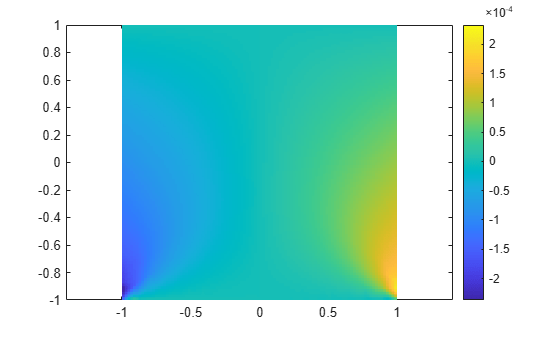Reshape the y-component of the normal strain to the shape of the grid and plot it.

eyy = reshape(intrpStrain.eyy,size(Y));
figure
py = pcolor(X,Y,eyy);
py.EdgeColor='none';
colorbar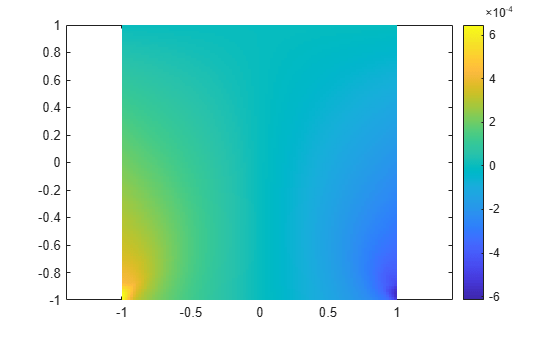Solve a static structural model representing a bimetallic cable under tension, and interpolate strain on a cross-section of the cable.

Create a static structural model for solving a solid (3-D) problem.

structuralmodel = createpde('structural','static-solid');

Create the geometry and include it in the model. Plot the geometry.

gm = multicylinder([0.01,0.015],0.05);
structuralmodel.Geometry = gm;
pdegplot(structuralmodel,'FaceLabels','on','CellLabels','on','FaceAlpha',0.5)Specify the Young's modulus and Poisson's ratio for each metal.

structuralProperties(structuralmodel,'Cell',1,'YoungsModulus',110E9, ...
'PoissonsRatio',0.28);
structuralProperties(structuralmodel,'Cell',2,'YoungsModulus',210E9, ...
'PoissonsRatio',0.3);

Specify that faces 1 and 4 are fixed boundaries.

structuralBC(structuralmodel,'Face',[1,4],'Constraint','fixed');

Specify the surface traction for faces 2 and 5.

Generate a mesh and solve the problem.

generateMesh(structuralmodel);
structuralresults = solve(structuralmodel)
structuralresults =
StaticStructuralResults with properties:

Displacement: [1x1 struct]
Strain: [1x1 struct]
Stress: [1x1 struct]
VonMisesStress: [22281x1 double]
Mesh: [1x1 FEMesh]

Define the coordinates of a midspan cross-section of the cable.

[X,Y] = meshgrid(linspace(-0.015,0.015,50));
Z = ones(size(X))*0.025;

Interpolate the strain and plot the result.

intrpStrain = interpolateStrain(structuralresults,X,Y,Z);
surf(X,Y,reshape(intrpStrain.ezz,size(X)))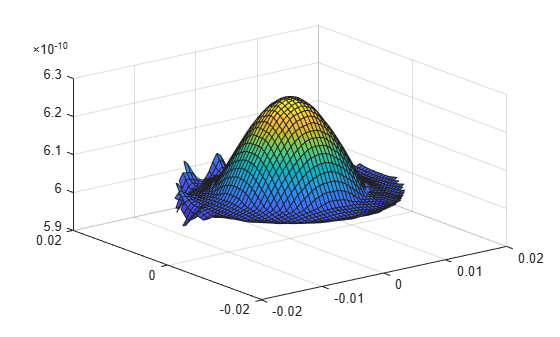Alternatively, you can specify the grid by using a matrix of query points.

querypoints = [X(:),Y(:),Z(:)]';
intrpStrain = interpolateStrain(structuralresults,querypoints);
surf(X,Y,reshape(intrpStrain.ezz,size(X)))Interpolate the strain at the geometric center of a beam under a harmonic excitation.

Create a transient dynamic model for a 3-D problem.

structuralmodel = createpde('structural','transient-solid');

Create a geometry and include it in the model. Plot the geometry.

gm = multicuboid(0.06,0.005,0.01);
structuralmodel.Geometry = gm;
pdegplot(structuralmodel,'FaceLabels','on','FaceAlpha',0.5)
view(50,20)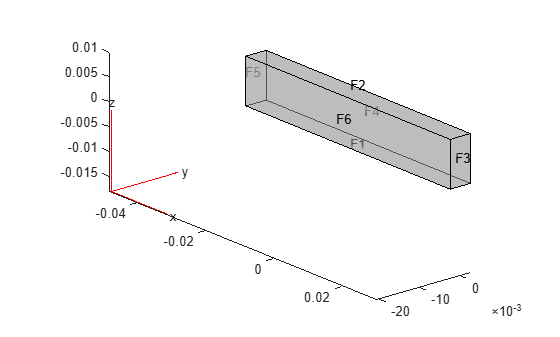Specify the Young's modulus, Poisson's ratio, and mass density of the material.

structuralProperties(structuralmodel,'YoungsModulus',210E9, ...
'PoissonsRatio',0.3, ...
'MassDensity',7800);

Fix one end of the beam.

structuralBC(structuralmodel,'Face',5,'Constraint','fixed');

Apply a sinusoidal displacement along the y-direction on the end opposite the fixed end of the beam.

structuralBC(structuralmodel,'Face',3,'YDisplacement',1E-4,'Frequency',50);

Generate a mesh.

generateMesh(structuralmodel,'Hmax',0.01);

Specify the zero initial displacement and velocity.

structuralIC(structuralmodel,'Displacement',[0;0;0],'Velocity',[0;0;0]);

Solve the model.

tlist = 0:0.002:0.2;
structuralresults = solve(structuralmodel,tlist);

Interpolate the strain at the geometric center of the beam.

coordsMidSpan = [0;0;0.005];
intrpStrain = interpolateStrain(structuralresults,coordsMidSpan);

Plot the normal strain at the geometric center of the beam.

figure
plot(structuralresults.SolutionTimes,intrpStrain.exx)
title('X-Direction Normal Strain at Beam Center')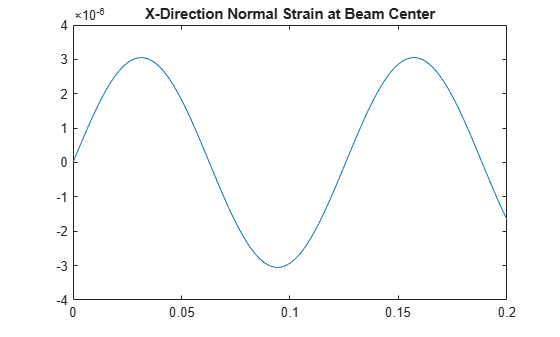Input Arguments

collapse all

Solution of the structural analysis problem, specified as a StaticStructuralResults, TransientStructuralResults, or FrequencyStructuralResults object. Create structuralresults by using the solve function.

Example: structuralresults = solve(structuralmodel)

x-coordinate query points, specified as a real array. interpolateStrain evaluates the strains at the 2-D coordinate points [xq(i),yq(i)] or at the 3-D coordinate points [xq(i),yq(i),zq(i)]. Therefore, xq, yq, and (if present) zq must have the same number of entries.

interpolateStrain converts query points to column vectors xq(:), yq(:), and (if present) zq(:). The function returns strains as a structure array with fields of the same size as these column vectors. To ensure that the dimensions of the returned solution are consistent with the dimensions of the original query points, use the reshape function. For example, use intrpStrain = reshape(intrpStrain.exx,size(xq)).

Data Types: double

y-coordinate query points, specified as a real array. interpolateStrain evaluates the strains at the 2-D coordinate points [xq(i),yq(i)] or at the 3-D coordinate points [xq(i),yq(i),zq(i)]. Therefore, xq, yq, and (if present) zq must have the same number of entries. Internally, interpolateStrain converts the query points to the column vector yq(:).

Data Types: double

z-coordinate query points, specified as a real array. interpolateStrain evaluates the strains at the 3-D coordinate points [xq(i),yq(i),zq(i)]. Therefore, xq, yq, and zq must have the same number of entries. Internally, interpolateStrain converts the query points to the column vector zq(:).

Data Types: double

Query points, specified as a real matrix with either two rows for 2-D geometry or three rows for 3-D geometry. interpolateStrain evaluates the strains at the coordinate points querypoints(:,i), so each column of querypoints contains exactly one 2-D or 3-D query point.

Example: For 2-D geometry, querypoints = [0.5,0.5,0.75,0.75; 1,2,0,0.5]

Data Types: double

Output Arguments

collapse all

Strains at the query points, returned as a structure array with fields representing spatial components of strain at the query points. For query points that are outside the geometry, intrpStrain returns NaN.

Introduced in R2017b

Partial Differential Equation Toolbox DocumentationGet trial now